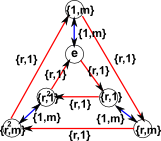# Maths - Bicrossed Product

### Direct Product

Perhaps the simplest way to combine (multiply) two sets (and therefore groups) is to use the Cartesian product as explained on this page. This product produces a set from the product:

g×h = {g, h}

So the result of this product is a different type of entity than the elements being multiplied, so the multiplication is not closed, and therefore does not represent a group. The external product makes this into a group because the inputs to the multiplication are also sets:

{y,z}{y',z' } = {y(z • y'),zy' z'}

where:

• × is the operation of the combined algebra.
• * is the operation of the group G.
• o is the operation of the group H which may be, or may not be, the same as *.

### Example C2×C3

In order to try to understand this product of two groups lets try multiplying two very simple groups together, the simplest groups I can think of are C2 and C3.

#### C2

generator cayley graph table permutation representation
<m | m²>1 m m 1
< ( 1 2 ) >
 0 1 1 0

#### C3

generator cayley graph table permutation representation
<r | r³>1 r r² r r² 1 r² 1 r
< ( 1 2 3 ) >
 0 0 1 1 0 0 0 1 0

#### direct product C3 × C2

This gives :

generator cayley graph table
<m,r | m²,r³,rm=mr>{1,1} {r,1} {r²,1} {1,m} {r,m} {r²,m} {r,1} {r²,1} {1,1} {r,m} {r²,m} {1,m} {r²,1} {1,1} {r,1} {r²,m} {1,m} {r,m} {1,m} {r,m} {r²,m} {1,1} {r,1} {r²,1} {r,m} {r²,m} {1,m} {r,1} {r²,1} {1,1} {r²,m} {1,m} {r,m} {r²,1} {1,1} {r,1}
permutation representation
<(1 2 3)(4 5 6),(1 4)(2 5)(3 6)>
[
 0 0 1 0 0 0 1 0 0 0 0 0 0 1 0 0 0 0 0 0 0 0 0 1 0 0 0 1 0 0 0 0 0 0 1 0
,
 0 0 0 1 0 0 0 0 0 0 1 0 0 0 0 0 0 1 1 0 0 0 0 0 0 1 0 0 0 0 0 0 1 0 0 0
]

Note that, in addition to applying both the generators and constrains for the original groups we have had to apply an additional constraint: rm=mr. If we had not done this we would have the infinite free product.

## Generating a Bicrossed Product using a Program

We can use a computer program to generate these groups, here I have used Axiom/FriCAS which is described here.

 ` `The Princeton Companion to Mathematics - This is a big book that attempts to give a wide overview of the whole of mathematics, inevitably there are many things missing, but it gives a good insight into the history, concepts, branches, theorems and wider perspective of mathematics. It is well written and, if you are interested in maths, this is the type of book where you can open a page at random and find something interesting to read. To some extent it can be used as a reference book, although it doesn't have tables of formula for trig functions and so on, but where it is most useful is when you want to read about various topics to find out which topics are interesting and relevant to you.